Processing ......FreeComputerBooks.com Links to Free Computer, Mathematics, Technical Books all over the World

Numerical Methods with Applications, 2nd Edition
Top Free C Programming Books 🌠 - 100% Free or Open Source!
• Title Numerical Methods with Applications, 2nd Edition
• Author(s) Autar K Kaw, Egwu E Kalu, Duc Nguyen
• Publisher: lulu.com (March 7, 2011); eBook (Mathematice for College)
• Paperback 740 pages
• eBook PDF (758 pages)
• Language: English
• ISBN-10: 0578057654
• ISBN-13: 978-0578057651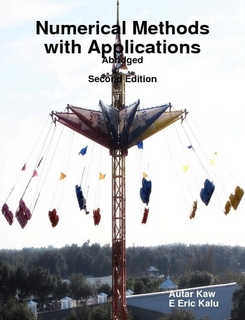Book Description

This book entitled Numerical Methods with Applications is written primarily for engineering undergraduates taking a course in Numerical Methods. The textbook offers a unique treatise to numerical methods which is based on a holistic approach and short chapters.

This book is a product of many years of work on educational projects funded since 2002 by the NSF. Features: 1) Examples of real-life applications are available from seven different engineering majors. 2) Each chapter is followed by multiple-choice questions. 3) Supplemental material such as primers on differential and integral calculus, and ordinary differential equations are available on the web. 4) The book has a state-of-art dedicated open courseware website with YouTube lectures, extra examples, PowerPoint presentations, worksheets in MATLAB, MATHEMATICA, Maple and MathCAD, anecdotes, eBooks, and blogs. To access the website, go to http://numericalmethods.eng.usf.edu

• N/A
Reviews, Ratings, and Recommendations: Related Book Categories: Read and Download Links:Similar Books:
•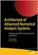Architecture of Advanced Numerical Analysis Systems

This unique open access book applies the functional OCaml programming language to numerical or computational weighted data science, engineering, and scientific applications. It's based on Owl, an OCaml-based numerical computing library.

•Tea Time Numerical Analysis: Experiences in Mathematics

This textbook was born of a desire to contribute a viable, free, introductory Numerical Analysis textbook. The ultimate goal of this book is to be a complete, one-semester, single-pdf, downloadable textbook designed for mathematics classes.

•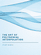The Art of Polynomial Interpolation (Stuart Murphy)

Exploring the techniques of interpolating data allows us to view the development and birth of a polynomial. This book is focused on laying a foundation for understanding and applying several common forms of Polynomial Interpolation.

•Numerical Algorithms: Computer Vision, Machine Learning, etc.

This book presents a new approach to numerical analysis for modern computer scientists, covers a wide range of topics - from numerical linear algebra to optimization and differential equations - focusing on real-world motivation and unifying themes.

•Numerical Methods and MATLAB Programming for Engineers

It covers the fundamentals while emphasizing the most essential numerical methods. Readers will enhance their programming skills using MATLAB to implement algorithms, discover how to use MATLAB to solve problems in science and engineering.

•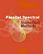Parallel Spectral Numerical Methods (Gong Chen, et al)

This book teaches the principals of Fourier spectral methods, their utility in solving partial differential equation and how to implement them in code. The programs will use Matlab and Fortran. A Python implementation of some of the Matlab programs is also provided.

•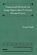Numerical Methods for Large Eigenvalue Problems (Yousef Saad)

This book is intended for researchers in applied mathematics and scientific computing as well as for practitioners interested in understanding the theory of numerical methods used for eigenvalue problems.

•First Semester in Numerical Analysis with Julia (Giray Okten)

This book presents the theory and methods, together with the implementation of the algorithms using the Julia programming language. The book covers computer arithmetic, root-finding, numerical quadrature and differentiation, and approximation theory.

•Numerical Analysis - Theory and Application (Jan Awrejcewicz)

This book is a book focused on introducing theoretical approaches of numerical analysis as well as applications of various numerical methods to either study or solving numerous theoretical and engineering problems.

•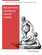High Performance Computing and Numerical Modelling

This textbook provides a step-by-step approach to numerical methods in engineering modelling. It provides a consistent treatment of the topic, from the ground up, to reinforce for students that numerical methods are a set of mathematical modelling tools.

•Numerical Computing with MATLAB (Cleve B. Moler)

This book is a lively textbook for an introductory course in numerical methods, MATLAB, and technical computing. It helps readers learn enough about the mathematical functions in MATLAB to use them correctly, and modify them appropriately, etc.

•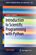Introduction to Scientific Programming with Python

This book offers an initial introduction to programming for scientific and computational applications using the Python programming language. The presentation style is compact and example-based, assuming little or no prior experience in programming.

Book Categories
 :All CategoriesTop Free BooksRecent BooksMiscellaneous BooksComputer EngineeringComputer LanguagesComputer ScienceData Science/DatabasesJava and Java EE (J2EE)Linux and UnixMathematicsMicrosoft and .NETMobile ComputingNetworking and CommunicationsSoftware EngineeringSpecial TopicsWeb Programming
Other Categories